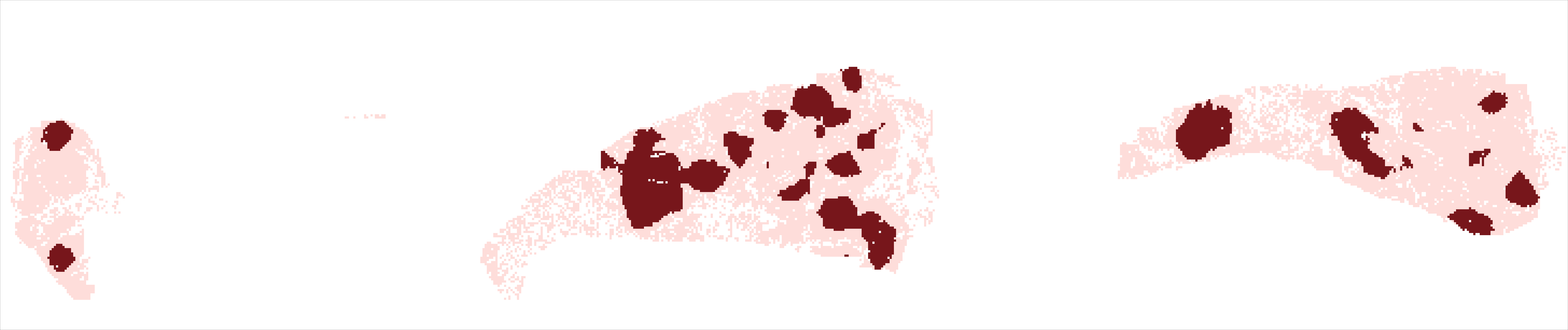# Beyond immune density: critical role of spatial heterogeneity in oestrogen receptor-negative breast cancer

Nawaz et al., Feb 2015

## Preparation

Click here to download the data for our study, including cell type and location information for all 245 breast tumours, as well as the hotspot analysis function. After download, load it into R, and initiate the required R libraries to proceed.

``````library(survival)
library(clinfun)
library(spdep)
``````

### Clinical information

The data file contains an object called trait', which is a data frame consisting of various clinical parameters as well as our hotspot analysis results. Each patient has a unique ID and is allocated to either the discovery or the validation cohort depending on their hospital site, given under the column site. The most important clinical parameter is S_10year which contains ten-year disease-specific survival information for every patient. Included also are: lymph node metastasis (node), tumour grade (grade), tumour size (size), tumour area (Area), lymphocytic abundance (lym), cancer and immune hotspot counts (cancer.hs and lympho.hs respectively), cancer-immune col-localisation as a fraction of cancer hotspot count (cancer.fractional.overlap), cancer-immune col-localisation as a fraction of immune hotspot count (immune.fractional.overlap), cancer-immune col-localisation as a fraction of tumour area analysed (normalised.overlap).

``````# Display clinical information data
``````
``````##   file site no.of.clhs.overlap cancer.fractional.overlap
## 1 1672    D                785                    0.2593
## 2 1680    D                  0                    0.0000
## 3 1690    D                397                    0.3265
## 4 1700    D                448                    0.6531
## 5 1704    D               1403                    0.4591
## 6 1714    D               1320                    0.4361
##   immune.fractional.overlap normalised.overlap squares cancer.hs lympho.hs
## 1                    0.4297            0.05499   14276      3027      1827
## 2                    0.0000            0.00000    2234       315       225
## 3                    0.3986            0.05415    7332      1216       996
## 4                    0.4595            0.02905   15424       686       975
## 5                    0.4536            0.05344   26254      3056      3093
## 6                    0.5036            0.04695   28113      3027      2621
##     time deaths      Area    lym node size grade S_10year
## 1 82.733      0 248000000 0.2053    0    1     3  82.733+
## 2 28.500      1  44000000 0.2006    1    2     3  28.500
## 3  8.067      0 116000000 0.2472    0    2     3   8.067+
## 4 85.133      0 248000000 0.1090    0    3    NA  85.133+
## 5 66.733      1 400000000 0.2972    0    2     3  66.733
## 6 78.700      0 331448000 0.2952    1    1     3  78.700+
``````

### Hotspots

The data file contains counts of cancer and immune hotspots for every patient as detected by Getis-Ord hotspot analysis. We can plot the distribution of cancer and immune hotspots in our discovery and validation cohorts.

``````# Separate discovery and validation cohorts
disc <- subset(trait, trait\$site == "D")
vald <- subset(trait, trait\$site == "V")
par(mfrow = c(2, 2))
hist(disc\$cancer.hs, breaks = 25, xlim = c(0, 8000), ylim = c(0, 20), font.lab = 2,
xlab = "No. of cancer hotspots", main = "Discovery cohort", col = "red",
cex.axis = 1.2, cex.lab = 1.4, cex.main = 1.8, bty = "l")
hist(vald\$cancer.hs, breaks = 25, xlim = c(0, 8000), ylim = c(0, 20), xlab = "No. of cancer hotspots",
main = "Validation cohort", col = "green", cex.axis = 1.2, cex.lab = 1.4,
cex.main = 1.8, font.lab = 2, bty = "l")
hist(disc\$lympho.hs, breaks = 25, xlim = c(0, 8000), ylim = c(0, 20), xlab = "No. of immune hotspots",
main = "", col = "red", cex.axis = 1.2, cex.lab = 1.4, cex.main = 1.8, font.lab = 2,
bty = "l")
hist(vald\$lympho.hs, breaks = 25, xlim = c(0, 8000), ylim = c(0, 20), xlab = "No. of immune hotspots",
main = "", col = "green", cex.axis = 1.2, cex.lab = 1.4, cex.main = 1.8,
font.lab = 2, bty = "l")
``````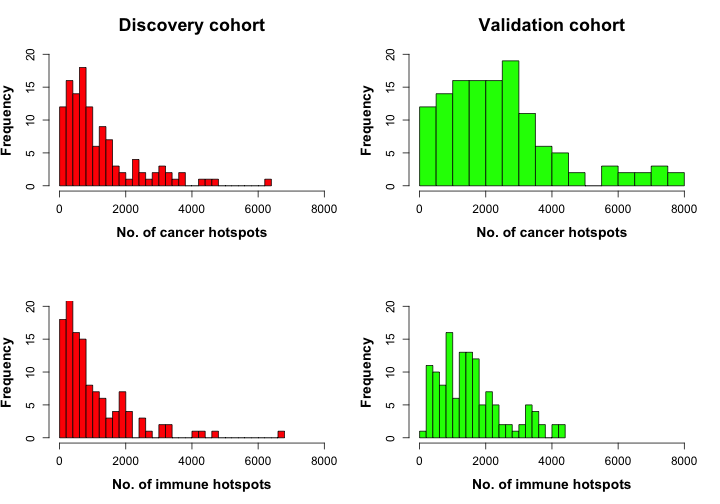## Clinical relevance of hotspots

### Prognostic value of hotspots

We can plot Kaplan-Meier curves to illustrate the prognostic value of hotspot co-localisation degree, by dividing patients into lower and higher co-localisation groups using a significant cut-off (0.0191) in normalised co-localisation.

``````# Look at survival information for discovery cohort
test.surv.d <- survdiff(S_10year ~ (normalised.overlap >= 0.0191), data = disc,
rho = 0)
test.cox.d <- summary(coxph(S_10year ~ (normalised.overlap >= 0.0191), data = disc))
test.surv.d
test.cox.d
# Look at survival information for validation cohort
test.surv.v <- survdiff(S_10year ~ (normalised.overlap >= 0.0191), data = vald,
rho = 0)
test.cox.v <- summary(coxph(S_10year ~ (normalised.overlap >= 0.0191), data = vald))
test.surv.v
test.cox.v
# Plot KM curves
rfit.disc <- survfit(S_10year ~ (normalised.overlap >= 0.0191), data = disc,
type = "kaplan-meier")
rfit.vald <- survfit(S_10year ~ (normalised.overlap >= 0.0191), data = vald,
type = "kaplan-meier")
par(mfrow = c(1, 2))
plot(rfit.disc, mark.time = T, bty = "n", col = c("purple", "brown"), lwd = 2,
xlab = "Time (months)", ylab = "Survival proportion", main = "Discovery cohort",
font.main = 2, font.lab = 2, cex.axis = 1.2, cex.lab = 1.4, cex.main = 1.8)
legend("bottomleft", cex = 1.2, col = c("purple", "brown"), legend = c(paste(c("C-I hotspots < 1.91%: ",
test.surv.d\$n[], "(", test.surv.d\$obs[], ")"), collapse = ""), paste(c("C-I hotspots > 1.91%: ",
test.surv.d\$n[], "(", test.surv.d\$obs[], ")"), collapse = "")), lwd = 3,
bty = "o")
legend("topright", legend = paste(c("p = ", round(test.cox.d\$waldtest[],
digits = 3)), collapse = ""), bty = "n", cex = 1.4)
plot(rfit.vald, mark.time = T, bty = "n", col = c("purple", "brown"), lwd = 2,
xlab = "Time (months)", ylab = "Survival proportion", main = "Validation cohort",
font.main = 2, font.lab = 2, cex.axis = 1.2, cex.lab = 1.4, cex.main = 1.8)
legend("bottomleft", cex = 1.2, col = c("purple", "brown"), legend = c(paste(c("C-I hotspots < 1.91%: ",
test.surv.v\$n[], "(", test.surv.v\$obs[], ")"), collapse = ""), paste(c("C-I hotspots > 1.91%: ",
test.surv.v\$n[], "(", test.surv.v\$obs[], ")"), collapse = "")), lwd = 3,
bty = "o")
legend("topright", legend = paste(c("p = ", round(test.cox.v\$waldtest[],
digits = 3)), collapse = ""), bty = "n", cex = 1.4)
``````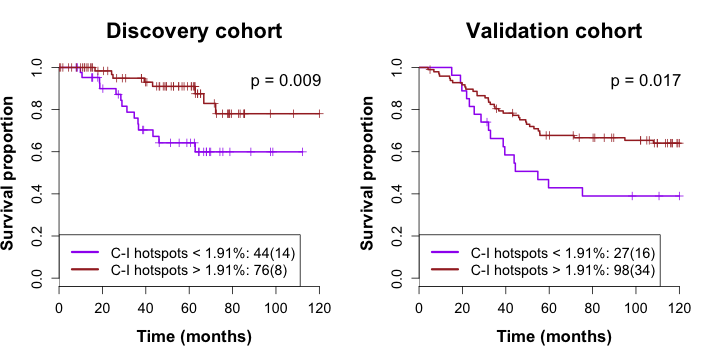The number of patients and deaths in each group is reported by the survdiff function, while the p-value of the difference between the groups in each cohort is evaluated using the Wald test in coxph. Coxph also gives us the hazard ratio for each group, including its 95\% confidence interval.

### Comparisons to known clinical parameters

To investigate the relationship between co-localised hotspots and known clinical parameters known to affect patient outcome, we first carry out some statistical tests. In the case of node metastasis and size, which are all categorical variables, we carry out the Jonkheere-Tersptra test to assess the strength of association between these and degree of hotspot co-localisation. For lymphocytic abundance and tumour area, we use Pearson's correlation test. An example of both tests follows:

``````jonckheere.test(disc\$normalised.overlap, disc\$node)
``````
``````##
##  Jonckheere-Terpstra test
##
## data:
## JT = 1886, p-value = 0.6881
## alternative hypothesis: two.sided
``````
``````cor.test(disc\$lym, disc\$normalised.overlap, method = "pearson")
``````
``````##
##  Pearson's product-moment correlation
##
## data:  disc\$lym and disc\$normalised.overlap
## t = 2.421, df = 119, p-value = 0.01699
## alternative hypothesis: true correlation is not equal to 0
## 95 percent confidence interval:
##  0.03969 0.38043
## sample estimates:
##    cor
## 0.2166
``````

We can then make box and scatter plots to illustrate these relationships.

``````# Make plots for discovery cohort patients
par(mfrow = c(2, 2), oma = c(0, 0, 3, 1))
for.box <- disc[, c("normalised.overlap", "node")]
rownames(for.box) <- NULL
boxplot(normalised.overlap ~ node, data = for.box, names = c("Absent", "Present"),
font.main = 2, cex.axis = 2, lwd = 2)
legend("topright", legend = "p = 0.688", bty = "n", cex = 2.2)
title(xlab = "Node metastasis", cex.lab = 2.2, font.lab = 2)
for.box <- disc[, c("normalised.overlap", "size")]
rownames(for.box) <- NULL
boxplot(normalised.overlap ~ size, data = for.box, cex.axis = 2, lwd = 2)
legend("topright", legend = "p = 0.584", bty = "n", cex = 2.2)
title(xlab = "Size", cex.lab = 2.2, font.lab = 2)
plot(disc\$lym, disc\$normalised.overlap, pch = 19, xlab = "Lym", ylab = "", cex.axis = 2,
cex.lab = 2.2, font.main = 2, font.lab = 2)
legend("topright", legend = "r = 0.212\np = 0.017", bty = "n", cex = 2.2)
plot(vald\$Area, vald\$normalised.overlap, pch = 19, xlab = "Area", ylab = "",
cex.axis = 2, cex.lab = 2.2, font.main = 2, font.lab = 2)
legend("topright", legend = "r = 0.172\np = 0.060", bty = "n", cex = 2.2)
mtext("Discovery cohort", side = 3, outer = TRUE, cex = 1.8, font.main = 2)
````````````# Make plots for validation cohort patients
par(mfrow = c(2, 2), oma = c(0, 0, 3, 1))
for.box <- vald[, c("normalised.overlap", "node")]
rownames(for.box) <- NULL
boxplot(normalised.overlap ~ node, data = for.box, ylim = c(0, 0.18), names = c("Absent",
"Present"), font.main = 2, cex.axis = 2, lwd = 2)
legend("topright", legend = "p = 0.682", bty = "n", cex = 2.2)
title(xlab = "Node metastasis", cex.lab = 2.2, font.lab = 2)
for.box <- vald[, c("normalised.overlap", "size")]
rownames(for.box) <- NULL
boxplot(normalised.overlap ~ size, data = for.box, cex.axis = 2, lwd = 2)
legend("topright", legend = "p = 0.106", bty = "n", cex = 2.2)
title(xlab = "Size", cex.lab = 2.2, font.lab = 2)
plot(vald\$lym, vald\$normalised.overlap, pch = 19, xlab = "Lym", ylab = "", cex.axis = 2,
cex.lab = 2.2, font.main = 2, font.lab = 2)
legend("topright", legend = "r = 0.237\np = 0.007", bty = "n", cex = 2.2)
plot(vald\$Area, vald\$normalised.overlap, pch = 19, xlab = "Area", ylab = "",
cex.axis = 2, cex.lab = 2.2, font.main = 2, font.lab = 2)
legend("topright", legend = "r = -0.068\np = 0.442", bty = "n", cex = 2.2)
mtext("Validation cohort", side = 3, outer = TRUE, cex = 1.8, font.main = 2)
``````### Additional prognostic value of co-localised hotspots to lymphocytic abundance

The degree of hotspot co-localisation can further stratify patients group according to high and low lymphocytic abundance as previously reported by Yuan et al. (2012). We can show that patients with high lymphocytic abundance may still face poor survival rates of hotspot co-localisation is low.

``````# Divide patients using the significant cut-offs in lym and co-localised
# hotspots (normalised.overlap)
data <- trait[, c("normalised.overlap", "lym")]
row.names(data) <- NULL
ll.lh <- subset(data, data\$lym < 0.0852 & data\$normalised.overlap < 0.0191)
ll.hh <- subset(data, data\$lym < 0.0852 & data\$normalised.overlap >= 0.0191)
hl.lh <- subset(data, data\$lym >= 0.0852 & data\$normalised.overlap < 0.0191)
hl.hh <- subset(data, data\$lym >= 0.0852 & data\$normalised.overlap >= 0.0191)

par(mfrow = c(2, 2))

# Plot survival curve for hotspots
test.surv.hs <- survdiff(S_10year ~ (normalised.overlap >= 0.0191), data = trait,
rho = 0)
test.cox.hs <- summary(coxph(S_10year ~ (normalised.overlap >= 0.0191), data = trait))
rfit <- survfit(S_10year ~ (normalised.overlap >= 0.0191), data = trait, type = "kaplan-meier")
plot(rfit, mark.time = T, bty = "n", col = c("red", "green"), lwd = 2, xlab = "Time (months)",
ylab = "Survival proportion", main = paste(c("Hotspots, p = ", round(test.cox.hs\$waldtest[],
4)), collapse = ""), font.main = 2, font.lab = 2, cex.axis = 1.2, cex.lab = 1.4,
cex.main = 1.8)
legend("bottomleft", cex = 1.2, col = c("green", "red"), legend = c(paste(c("High: ",
test.surv.hs\$n[], "(", test.surv.hs\$obs[], ")"), collapse = ""), paste(c("Low: ",
test.surv.hs\$n[], "(", test.surv.hs\$obs[], ")"), collapse = "")),
lwd = 3, bty = "o")

# Plot survival curve for lym
test.surv.lym <- survdiff(S_10year ~ (lym >= 0.0852), data = trait, rho = 0)
test.cox.lym <- summary(coxph(S_10year ~ (lym >= 0.0852), data = trait))
rfit <- survfit(S_10year ~ (lym >= 0.0852), data = trait, type = "kaplan-meier")
plot(rfit, mark.time = T, bty = "n", col = c("red", "green"), lwd = 2, xlab = "Time (months)",
ylab = "Survival proportion", main = paste(c("Lym, p = ", round(test.cox.lym\$waldtest[],
3)), collapse = ""), font.main = 2, font.lab = 2, cex.axis = 1.2, cex.lab = 1.4,
cex.main = 1.8)
legend("bottomleft", cex = 1.2, col = c("green", "red"), legend = c(paste(c("High: ",
test.surv.lym\$n[], "(", test.surv.lym\$obs[], ")"), collapse = ""),
paste(c("Low: ", test.surv.lym\$n[], "(", test.surv.lym\$obs[], ")"),
collapse = "")), lwd = 3, bty = "o")

# Plot the distribution
cor <- cor.test(trait\$lym, trait\$normalised.overlap)
plot(ll.lh, col = "red", pch = 19, xlim = c(0, 0.15), ylim = c(0, 0.5), cex.axis = 1.2,
lwd = 2, cex.main = 1.8, main = paste(c("r = 0.18", ", p = ", signif(cor\$p.value,
1)), collapse = ""), xlab = "Hotspots", ylab = "Lym", cex.lab = 1.4,
font.lab = 2)
points(ll.hh, col = "blue", pch = 19)
points(hl.lh, col = "green", pch = 19)
points(hl.hh, col = "orange", pch = 19)
abline(v = 0.0191, lwd = 2, lty = 2)
abline(h = 0.0852, lwd = 2, lty = 2)

# Plot survival curve for hotspots and lym
test.surv.both <- survdiff(S_10year ~ (normalised.overlap > 0.0191) + (lym >
0.085), data = trait, rho = 0)
test.cox.both <- summary(coxph(S_10year ~ (normalised.overlap > 0.0191) + (lym >
0.085), data = trait))
f <- survfit(S_10year ~ (normalised.overlap > 0.0191) + (lym > 0.085), data = trait,
type = "kaplan-meier")
plot(f, col = c("red", "green", "blue", "orange"), lwd = 2, bty = "n", xlab = "Time (months)",
ylab = "Survival proportion", main = paste(c("Combined p = 0.0014"), collapse = ""),
font.main = 2, font.lab = 2, cex.axis = 1.2, cex.lab = 1.4, cex.main = 1.8)
legend("bottomleft", cex = 1, col = c("orange", "blue", "green", "red"), legend = c(paste(c("High lym & high hotspots: ",
test.surv.both\$n[], "(", test.surv.both\$obs[], ")"), collapse = ""),
paste(c("Low lym & high hotspots: ", test.surv.both\$n[], "(", test.surv.both\$obs[],
")"), collapse = ""), paste(c("High lym & low hotspots: ", test.surv.both\$n[],
"(", test.surv.both\$obs[], ")"), collapse = ""), paste(c("Low lym & low hotspots: ",
test.surv.both\$n[], "(", test.surv.both\$obs[], ")"), collapse = "")),
lwd = 3, bty = "o")
``````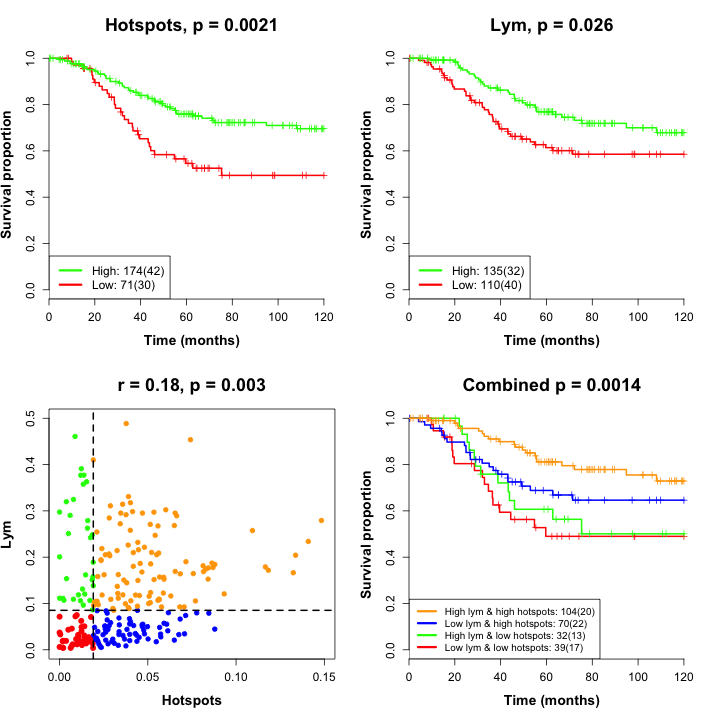In a previous paper (Yuan et al., 2012), we had reported a significant threshold in lym of 8\%. However, in this dataset, 8\% is not the optimum threshold, as can be seen from the next figure.

``````# Plot survival curves for each group
test.surv.oldlym <- survdiff(S_10year ~ (lym >= 0.08), data = trait, rho = 0)
test.cox.oldlym <- summary(coxph(S_10year ~ (lym >= 0.08), data = trait))
rfit <- survfit(S_10year ~ (lym >= 0.08), data = trait, type = "kaplan-meier")
plot(rfit, mark.time = T, bty = "n", col = c("red", "green"), lwd = 2, xlab = "Time (months)",
ylab = "Survival proportion", main = paste(c("Lym, p = ", signif(test.cox.oldlym\$waldtest[],
2)), collapse = ""), font.main = 2, font.lab = 2, cex.axis = 1.2, cex.lab = 1.4,
cex.main = 1.8)
legend("bottomleft", cex = 1.2, col = c("green", "red"), legend = c(paste(c("High lym: ",
test.surv.oldlym\$n[], "(", test.surv.oldlym\$obs[], ")"), collapse = ""),
paste(c("Low lym: ", test.surv.oldlym\$n[], "(", test.surv.oldlym\$obs[],
")"), collapse = "")), lwd = 3, bty = "o")
``````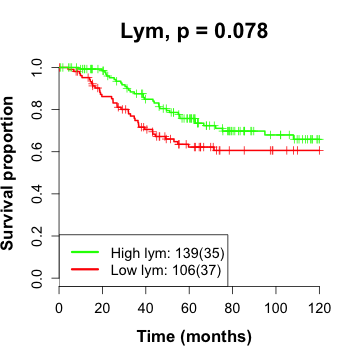The p-values for the Kaplan-Meier curves were found using the survdiff function.

## Threshold selection

### Discovery

We used the following method to objectively select the optimum threshold for differentiating between good and poor survival outcome in out discovery cohort patients.

``````steps <- 10000
no.value <- c()
nop.value <- c()
# Specify quantile range
lq <- 0.15
uq <- 0.85
n.q <- quantile(disc\$normalised.overlap, c(lq, uq))

count <- floor(as.numeric(signif(n.q, 3)/(1/steps)))
for (i in 1:count) {
no.value[i] <- i/steps
a <- summary(coxph(S_10year ~ (normalised.overlap < no.value[i]), data = disc))
nop.value[i] <- a\$coefficients
}
result.no <- as.data.frame(cbind(no.value, nop.value))
``````

We can then find a cut-off with the lowest p-value, and thus highest significance as a prognostic indicator in our discovery set.

``````result.no\$no.value[which(result.no\$nop.value == min(result.no\$nop.value))]
``````
``````##  0.0191
``````

### Validation

To check that the threshold found in the discovery set holds true in the validation set, we perform a simple survival analysis using survdiff.

``````summary(coxph(S_10year ~ (normalised.overlap >= 0.0191), data = vald))
``````
``````## Call:
## coxph(formula = S_10year ~ (normalised.overlap >= 0.0191), data = vald)
##
##   n= 125, number of events= 50
##    (4 observations deleted due to missingness)
##
##                                    coef exp(coef) se(coef)     z Pr(>|z|)
## normalised.overlap >= 0.0191TRUE -0.725     0.484    0.304 -2.38    0.017
##
## normalised.overlap >= 0.0191TRUE *
## ---
## Signif. codes:  0 '***' 0.001 '**' 0.01 '*' 0.05 '.' 0.1 ' ' 1
##
##                                  exp(coef) exp(-coef) lower .95 upper .95
## normalised.overlap >= 0.0191TRUE     0.484       2.06     0.267     0.879
##
## Concordance= 0.566  (se = 0.029 )
## Rsquare= 0.04   (max possible= 0.974 )
## Likelihood ratio test= 5.14  on 1 df,   p=0.0234
## Wald test            = 5.68  on 1 df,   p=0.0171
## Score (logrank) test = 5.93  on 1 df,   p=0.0149
``````

We can see that the reported p-value by the Wald test for this cut-off in our validation cohort is 0.0171 and therefore within the limit of significance.

## Running Getis-Ord hotspot analysis

### Description

Included in the supplementary material for our paper is an R function that contains an algorithm for Getis-ord hotspot detection. The primary function in this algorithm is “localG” and is available in the “spdep” package. Some other spdep functions, as well as our own functions are also present. The algorithm consists of three parts:

1. Evaluation of tissue cover in an image
2. Hotspot detection
3. Putting results in a table together with clinical data

The input of the algorithm is the result from cancer and lymphocyte detection via CRImage, followed by accumulation of all nuclei locations into a table using getCellPos (available as a separate function). Both of these are available as .rdata files titled CellPosAndMask for each image.

Tissue cover evaluation works by applying a virtual grid of a user-specified square size and using a binary mask (available in the .rdata file), which states whether or not a pixel is part of tissue, to calculate how many pixels in each grid square are part of tissue. If more than 50% of the pixels in a square are part of tissue, then the square is labelled as part of tissue and therefore counted. The user can choose whether to use the 50% value or some other value. The output of this part of the algorithm is the percentage of squares in the entire grid that are part of tissue.

Next, the same grid is again applied over the image and the cell counts are totalled. Only those images with minimum user-specified tissue cover and only those grid squares with minimum user-specified tissue cover are included. A neighbours list is constructed using “nb2listw” function in “spedep” and cell counts in the neighbouring area of user-specified radius are also totalled. These cell counts are then compared to the image mean and a z score is evaluated using the “localG” function. The output of this part is an .rdata file per image containing z scores for different cell types as well as key parameters such as square and neighbourhood size chosen.

The final part goes through each of the .rdata files generated by part 2 and counts the number of grid squares with z scores high enough to be classified as hotspots. The critical z score varies depending on the number of squares in the image grid, and this is taken into account. The hotspot counts (“cancer.hs” and “immune.hs”) per image are put into a table together with the clinical data. The function also evaluates per image the number of cancer-immune hotspots (“no.of.clhs.overlap”) and our three statistics, cancer fractional overlap, immune fractional overlap and cancer-immune hotspot overlap normalised by number of grid squares.

There is also an optional algorithm provided to generate heatmaps illustrating cancer, immune and cancer-immune overlapping hotspots. This does not run by default, but can be enabled by removing the hashtag (#) at the beginning of line 16 of the code.

### Example run

To begin, download and extract our data and R function from the link at the top. This data consists of a folder named “CellPos” which contains .rdata files for every image, as well as a separate clinical data file named “trait”. The files in CellPos contain type and location of every cell nucleus detected by CRImage, as well as a binary tissue map.

Next, load the function into R using the “source()” and set the correct working directory using “setwd('path/to/working/directory/)”. This is where results will be stored.

To execute the code, run the following command in R:

``````getisord(s = 10, n = 4, ptc = 10, ptc.persquare = 50, CellPos.path = "./data/CellPos/")
``````

The command “getisord” executes a chain of functions described in the previous section. There are five input parameters that are chosen by the user. The first, s, is the grid square size in pixels. The second, n, is the neighbourhood size in pixels. The third, ptc, is the minimum tissue cover percentage an image must have to be selected for analysis, and fourth, ptc.persquare, is the minimum percentage tissue cover a grid square must have to be a hotspot candidate. Last, the path to each of the CellPos folder is required.

The function creates a folder named “result” in the working directory. This folder contains a .txt file, e.g. “pc.cover_s10.txt” in the case of s=10, which list the percentage tissue cover of all the images for a given square size. A folder named “getisord_perimage” will also be created and contain hotspot analysis results as .rdata files for each image. The main output of the function is a .txt file beginning “hotspotsdata” followed by square and neighbourhood sizes in the suffix, e.g. “hotspotsdata_s10_n.txt”. Having run the analysis on one image for example, we can view what this looks like by loading it into R:

``````result <- read.delim("./result/hotspotsdata_s10_n4.txt", header = T)
``````
``````## Warning: incomplete final line found by readTableHeader on
## './result/hotspotsdata_s10_n4.txt'
``````
``````result
``````
``````##   file squares cancer.hs lympho.hs no.of.clhs.overlap
## 1 5343   24545      4360      1634                330
##   cancer.fractional.overlap immune.fractional.overlap normalised.overlap
## 1                   0.07569                     0.202            0.01344
##    time deaths      Area     lym node size grade site  S_10year
## 1 15.07      0 345666000 0.09649    1    2     3    D 15.06667+
``````

To generate heatmaps, remove the hashtag from line 16 and, if needed, insert it at the beginning of lines 7, 10 and 13 to avoid repeating the above steps. This part of the function will create a “hotspot_maps” folder in the “results” folder and contain folders with heatmap images as .png files organised in folder according to the square and neighbourhood sizes chosen. The top part of the function file should be as follows:

``````getisord <- function(s, n, ptc, ptc.persquare, CellPos.path) {

if (file.exists("result") == FALSE) {
dir.create("result")
} else {
}

# evaluate percentage tissue cover of every image tissue.cover <-
# evaluateCover(s,ptc.persquare,CellPos.path)

# get zscores getzscores(s,n,ptc,ptc.persquare,CellPos.path)

# generate results file results <- gather.results(s,n)

# plot hotspot heatmaps
plotHotspots(s, n)

}
``````

You will obtain the heatmaps below, with cancer hotspots in red, immune hotspots in blue and cancer-immune hotspots in green.